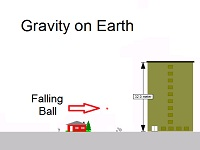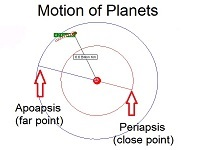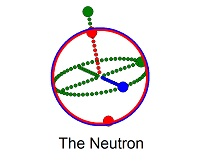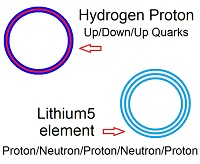# Axioms and Forces

Mass attracts itself (gravity) and energy repels itself (dark energy). Mass is confined energy and energy travels at the speed of light. Here we use a fixed grid and fixed timesteps, similar to frames in a movie, to model the particles and forces of mass and energy.

Animated physics is used to understand the properties of the real world. Once the forces and objects acting in the grid are quantified in space and time, they can be tested to see if they match reality.

## The axioms of Animated Physics

1.   Mass is attracted to itself (gravity)
2.   Mass is confined energy and energy travels at the speed of light
3.   Energy is repelled by itself (dark energy)

#### 1.   Mass is attracted to itself (called gravity)

Newton’s laws are used to simulate the motion of objects on the surface of planets, satellites travelling through space and planetary orbits around the sun.The force of attraction between two objects with mass is inversely proportional to the square of the distance between them.

With traditional physics, Newton’s force laws allow gravity to be simulated, but start to fail both on the very large scale and on the very small scale. Specifically, the orbit of Mercury cannot be accurately predicted over time with these calculations. In fact, using the inverse square law implies that no two body orbit will have a “precession”, yet many are observed.

#### 2.   Mass is confined energy and energy travels at the speed of lightEinstein’s relativity (based on the fixed speed of energy and the relationship between mass and energy) is used to allow for the orbit of Mercury to show its proper precession.

The fixed speed of light implies gravitational red shifting, observed elongation of something travelling at close to the speed of light, and different rates of time for observers in motion relative to each other. The fixed speed of energy also predicts frame dragging where mass is accelerated near a fast moving mass in the direction of the moving mass.

#### 3.   Energy is repelled by itself (called dark energy)Dark energy (or energy fluctuations at a specific point over time) repels itself in the same way as a wave spreads out over time.

The repulsive force of energy allows the calculation of the distribution and structure of the charge and magnetic fields on the neutron, proton and electron. This provides a basis of their quantum charge, spin and angular momentum values observed. Insight into the properties of matter and energy leads to the modelling of larger scale structures of particles and the complex world we can build from these particles.

## The Forces of Animated Physics

1.   The Strong Interaction
2.   Electro-Magnetism
3.   The Weak Interaction
4.   Gravity

#### 1.   The Strong Interaction

•Holds quarks together to form Protons and Neutrons
• Holds Protons and Neutrons together to form Elements (called the nuclear force that is 100 times stronger than the electromagnetic force)

The internal structure of the proton or neutron is made up of three quark layers. All baryons including the proton and neutron consist of three quarks. Mesons are made up of two quarks and are created in high speed collisions between baryons.

Elements are made from layers of proton shells separated with neutron shells (some extra neutron shells may exist). The name and charge of the element is determined by the number of protons. The mass of the element is determined by the total number of protons and neutrons.

#### 2.   Electro-Magnetism

Electrons are attracted to a proton as free electrons under coulomb’s law until they are close to a proton where they become trapped in orbitals of a specific energy level through the emission of a photon. This trapping of electrons in orbitals provides the structure to build bigger and more complex molecules. The properties of solids and liquids are determined by the structural layout of the electrons and elements.

Magnetism caused by the movement of charge has a significant effect on electrons both in their motion through space and in the electrons orientation in space. In this way, electrons can be accelerated and directed to form such things as television images.

#### 3.   The Weak Interaction

Energy in the form of photons or gamma rays can emit from a particle when the particle undergoes specific changes. Particles can be transformed and new ones created through instability in a particle.

#### 4.   Gravity

Collapsing energy can become so intense that nothing can escape it as in a black hole.